telescopeѲptics.net          ▪▪▪▪                                             CONTENTS

# 3.5.2. (cont.) Zernike aberration coefficients

Since both, standard aberration functions and Zernike aberration polynomials, are describing the same wavefront deviations, they can be related and converted from one form to the other.

Denoting Zernike aberration terms - the orthonormal Zernike polynomials - simply as Za (full designation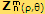, usually written as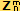or, in a single-index notation, as Zj, informally - and incorrectly - referred to as "Zernike coefficients"), and the corresponding Zernike expansion coefficients as za (usually written as cnm, here znm), where the term subscript a identifies the corresponding aberration as balanced primary spherical (S), coma (C) or astigmatism (A), Zernike polynomial form for these three point-image quality primary aberrations can be related to the peak aberration coefficients S, C and A from Eq. 5.1 as follows:

- spherical aberration:    ZS zS5(6ρ4-6ρ2+1)  =  |S|(6ρ4-6ρ2+1)/6,   with n=4, m=0, thus ZS=Zand zS=z40
- coma:    Z
CzC8(3ρ3-2ρ)cosθ  =  |C|(3ρ3-2ρ)cosθ/3,   with n=3, m=1, thus ZC=Z, and zC=z31
- astigmatism:     Z
A= zA24(cos2θ-0.5)ρ2  =  |A|(cos2θ-0.5)ρ2  with n=2, m=2, thus ZA=Zand zA=z22

with "||" indicating absolute - i.e. w/o numerical sign - value (note that the sign of m superscript and corresponding cosine function are according to the angle convention used on this site)

This implies that Zernike expansion coefficients zS, zC and zA, equal the corresponding RMS wavefront error ω, which is in terms of the peak aberration coefficient given by ωS=|S|/65=|S|/180, ωC=|C|/38=|C|/72 and ωA=|A|/24 for spherical aberration, coma and astigmatism, respectively. With respect to the Zernike aberration term, ωS=zS=ZS/5, ωC=zC=ZC/8 and ωA=zA=ZA/6 (coma and astigmatism along the ais of aberration, i.e. for θ=0).

Note that the RMS wavefront error is by definition numerically positive, unlike peak/P-V wavefront error, or Zernike expansion coefficients, which can be numerically negative.

Similarly, conversion for defocus is ZDzD3(2ρ2-1)= Pρ2/2, with n=2, m=0, thus ZD=Zand zD=z20 implying ωD=zD=ZD/23.

Note that these relations are for best focus location; also, in order for the nominal error to reflect its actual effect on diffraction intensity distribution, expressing the expansion coefficients as representing the RMS wavefront error requires the latter to be nearly identical to the phase factor φ of standard deviation, i.e. phase error averaged over the pupil (requirement fulfilled for low-level aberrations affecting most or all of wavefront area, roughly below λ/2 P-V in magnitude).

The above relations are valid for clear aperture (Zernike circle polynomials/coefficients). To an aperture with central obstruction applies different polynomial form (Zernike annular polynomials/coefficients). In this case, all three - RMS wavefront error, Zernike expansion coefficient and Zernike aberration term change according to a factor appropriate to each aberration form. Specifically,

ωSo= zSo = ZSo/5 = ωS(1-o2)2 = zS(1-o2)2= ZS(1-o2)2/5

ωCo= zCo = ZCo/8 = ZC(1-o2)(1+4o2+o4)1/2/8(1+o2)1/2  and

ωAo= zAo= ZAo/6 = ZA(1+o2+o4)1/2/6

for primary spherical aberration, coma and astigmatism, respectively, with o being the relative obstruction size in units of the aperture.

Following table gives an overview of the Zernike aberration forms for the most common monochromatic aberrations in telescopes, for clear circular aperture (aberrations in aperture with central obstruction are described with Zernike annular polynomials). The three point-image aberrations, spherical, coma and astigmatism, are balanced, with "balanced" as before, referring to the principal aberration form that combines two or more secondary aberrations in order to reduce error to a minimum (i.e. to the level at its diffraction, or best focus).

For instance, balanced primary spherical includes its principal aberration term ρ4 and balancing defocus term ρ2, coma includes its principal aberration term ρ3 and balancing tilt term ρ, secondary spherical, also in its balanced form (minimized by combining it with 4th order spherical and defocus, thus here referred to as balanced 6th/4th order spherical aberration, in order to distinguish it from balanced pure 6th order aberration, which is minimized by combining it with defocus alone) includes its principal aberration term ρ6 and two balancing terms, for lower-order spherical and defocus (ρ4 and ρ2, respectively). The polynomial forms are as given by Mahajan (Optical Imaging and Aberrations).

 ZERNIKE CIRCLE POLYNOMIALS FOR COMMON ABERRATIONS (BEST FOCUS LOCATION) I II III IV V VI ABERRATION n m ORTHOGONAL POLYNOMIAL P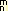(ρ,θ)=V(ρ)cos(mθ)=P ORTHONORMAL POLYNOMIAL P'=NP N=[2(n+1)/(1+δm0)]0.5 ZERNIKE ABERRATION TERM Z(ρ,θ)=P'znm=NPznm=Z RMS ERROR (EXPANSION COEFFICIENT) ωz=znm =Z/N Tilt (Distortion) 1 1 ρcosθ 2ρcosθ [2ρcosθ]z11 Ztd/2 Defocus (Field curvature) 2 0 2ρ2-1 √3(2ρ2-1) [√3(2ρ2-1)]z20 Zd /√3 Spherical primary (best focus) 4 0 6ρ4-6ρ2+1 √5(6ρ4-6ρ2+1) [√5(6ρ4-6ρ2+1)]z40 Zs1/√5 secondary (balanced 6th/4th) 6 0 20ρ6-30ρ4+12ρ2-1 √7(20ρ6-30ρ4+12ρ2-1) [√7(20ρ6-30ρ4+12ρ2-1)]z60 Zs2 /√7 Coma primary 3 1 (3ρ3-2ρ)cosθ √8(3ρ3-2ρ)cosθ [√8(3ρ3-2ρ)cosθ]z31 Zc1/√8 secondary 5 1 (10ρ5-12ρ3+3ρ)cosθ √12(10ρ5-12ρ3+3ρ)cosθ [√12(10ρ5-12ρ3+3ρ)cosθ]z51 Zc2/√12 Astigmatism primary 2 2 ρ2cos2θ √6ρ2cos2θ [√6ρ2cos2θ]z22 Za1/√6 secondary 4 2 (4ρ4-3ρ2)cos2θ √10(4ρ4-3ρ2)cos2θ [√10(4ρ4-3ρ2)cos2θ]z42 Za2/√10

TABLE 5: Zernike circle polynomials for selected balanced (best focus) aberrations.
COLUMN I
; "primary" refers to the lower, or 4th order wavefront aberration form, with n+m=4, and "secondary" to the subsequent higher, or 6th order form, with n+m=6 (in terms of ray aberrations, 3rd and 5th order, respectively);
COLUMN II
: integers specifying Zernike mode; the subscript n, or radial order, is the highest exponent of ρ in the radial variable (analog to the exponent of normalized pupil height factor in the standard aberration function); superscript m specifies angular frequency of the mode, with positive integers - such as those given in the table - indicating change in deviation with cosine of the angular coordinate θ, and negative integers indicating its change with sine function (numerically, m is analog to the radial power in image space for standard aberration functions);
COLUMN III
: General (orthogonal) form of the Zernike circle polynomial,
V(ρ)cos(mθ), is a product of its radial variable V(ρ) and angular variable cos(mθ);
COLUMN IV
: Orthonormal Zernike polynomial, normalized with a factor
N=[2(n+1)/(1+δm0)]1/2 that scales it to unit variance; in effect, the normalizing factor N scales the value of the polynomial to its RMS error level, which makes polynomial values for different aberrations directly comparable, as well as additive/subtractive; it is directly related to the manner in which zero mean splits the wavefront: as FIG. 32 illustrates, with balanced primary spherical aberration, the P-V wavefront error split relative to zero mean is proportional to (1+0.5), with the P-V/RMS ratio given by 1.55; for balanced secondary, the split is even, proportional to (1+1), with the P-V/RMS ratio given by 27 (FIG. 37C); for tilt, defocus, primary coma and astigmatism (last three, FIG.30-1), the wavefront
P-V split vs. zero mean is proportional to (1+1) and the P-V/RMS factor is given by 4, 2
3, 28 and 26, respectively  (δm0 in the normalization factor N is Kronecker delta of m and 0, thus δm0=0 for m≠0 and δm0=1 for m=0);
COLUMN V
: Zernike aberration term, or mode,
Z(ρ,θ), a product of the orthonormal polynomial P' and Zernike expansion coefficient znm, the latter equaling the RMS wavefront error in units of wavelength (the aberration term P' is what is popularly called "Zernike coefficient" value given by raytrace programs);
COLUMN VI
: Relative (in units of wavelength) RMS wavefront error
ωz corresponding to the Zernike aberration term; it is called  Zernike expansion coefficient, and obtained directly from the Zernike aberration term Z as ωz=znm=Z/NP, where coordinates for the orthogonal polynomial P=P(ρ,θ) are set to ρ=1 and θ=0 (thus giving 1 for any orthogonal polynomial P), resulting in ωz=znm=Z/N; denoting Z as a general Zernike aberration term , and terms with subscripts t, d, s1, s2, c1, c2, a1 and a2 as specifically referring to tilt, defocus, primary and secondary spherical, coma and astigmatism, respectively, the RMS error is obtained directly from the term value as indicated (obviously, any specific RMS error can be directly assigned the corresponding Zernike aberration term as Z=Nωz; for instance, for the diffraction limited RMS ω=1/√180, the corresponding Zernike term value for balanced lower-order spherical aberration is Zs1=Z=√5ω=√5z40=1/6).

Each separate polynomial in the above table describes single aberration of a perfect conical surface, hence only a single polynomial suffices to describe it; since the aberrations are separated, the wavefront orientation is inconsequential for describing the mode of deformation, and all radially non-symmetrical aberrations are given with a positive m integer (i.e. with cosine function in the angular variable).

As mentioned on page top, Zernike aberrations for specific telescope systems, or a mirror, are commonly given in the form of Zernike aberration term, which is, as illustrated on FIG. 31, a product of Zernike orthonormal (normalized to unit variance) polynomial and Zernike expansion coefficient (in effect the RMS wavefront error in units of the wavelength). While formally there is no limit to the number of these terms - or modes - that can be used to describe wavefront structure, relatively smooth wavefronts typically produced by telescope optics are well described by a limited number of Zernike modes. The terms are routinely referred to as "Zernike coefficients" by the amateurs (not seldom, informally, by non-amateurs as well), which is formally incorrect. Zernike (expansion) coefficient is a part of Zernike term; the coefficient equals the RMS error, for optical systems usually given in units of wavelength.

An expanded set of Zernike polynomials includes any chosen number of higher-order terms, in addition to the lower-order terms; in raytracing reports, they are often given in the form of a simple designation zi, with the subscript i indicating term's ordering number, and referred to as Zernike coefficients. This notation is inappropriate, since given values are for the Zernike term, which is always denoted by a capital letter, as opposed to the Zernike (expansion) coefficient - nominally equaling the RMS wavefront error - which is denoted with a small letter.

The first term is always piston - an aberration term associated with chief ray, which only constitutes an aberration in systems with two or more pupils differing in phase. It is normally followed by terms expressing lower-order aberrations, and then those for higher-order forms. Every aberration term except those with full rotational symmetry (defocus and spherical aberration) has two forms, one with cosine, and the other with sine of θ. The sine form effectively rotates wavefront pattern by 90/m degrees counterclockwise (m being, as before, the determinant of angular meridional frequency of the Zernike wavefront mode) with respect to its cosine form, producing wider variety of shapes needed to model asymmetric wavefronts.

Specific terms in a set of Zernike polynomials can vary, according to its purpose. Order of terms (or term expansion) is based on the polynomial ordering number. This number is used for a simplified, single-index term notation. There are several different definitions of the ordering number, with somewhat different forms of term expansion. For evaluating optical systems, one that is commonly used is based on the set of Zernike coefficients defined by J. Wyant (used in OSLO raytracing software). The full set lists 48 Zernike terms; these are the first 15:

TABLE 6: WYANT ZERNIKE TERMS EXPANSION (first 15) AND RELATION TO STANDARD ABERRATIONS

 1 2 3 4 5 6 # ZERNIKE TERM (often erroneously referred to as coefficient) Zernike ORTHOGONAL circle polynomial RMS ERROR (Zernike coefficient's absolute value) Aberration WF Map w/ANSI sign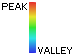Name Standard  aberration function Paraxial focus Best focus *deviation from zero mean 0 Z0 1 Z0 piston - -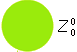1 Z1 ρcosθ Z1 /2 distortion/tilt ρcosθ ρcosθ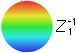2 Z2 ρsinθ Z2 /2 - - 3 Z3 2ρ2-1 Z3 /√3 defocus/field curvature ρ2 ρ2 ρ2-0.5*4 Z4 ρ2cos2θ Z4 /√6 primary astigmatism ρ2cos2θ ρ2(cos2θ-0.5) *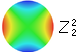5 Z5 ρ2sin2θ Z5 /√6 - - 6 Z6 (3ρ3-2ρ)cosθ Z6 /√8 primary coma ρ3cosθ * (ρ3-2ρ/3)cosθ *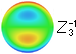7 Z7 (3ρ3-2ρ)sinθ Z7 /√8 - - 8 Z8 6ρ4-6ρ2+1 Z8 /√5 BALANCED primary spherical aberration ρ4 ρ4-ρ2 ρ4-ρ2+1/6 *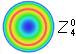9 Z9 ρ3cos3θ Z9 /√8 elliptical coma (ARROWS, TREFOIL) ρ3cos3θ * ρ3cos3θ *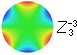10 Z10 ρ3sin3θ Z10 /√8 - - 11 Z11 (4ρ4-3ρ2)cos2θ Z11 /√10 secondary astigmatism ρ4cos2θ (ρ4-0.75ρ2)cos2θ *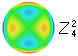12 Z12 (4ρ4-3ρ2)sin2θ Z12 /√10 - - 13 Z13 (10ρ5-12ρ3+3ρ)cosθ Z13 /√12 secondary coma ρ5cosθ (ρ5-1.2ρ3+0.3ρ)cosθ *14 Z14 (10ρ5-12ρ3+3ρ)sinθ Z14 /√12 - - 15 Z15 20ρ6-30ρ4+12ρ2-1 Z15 /√7 secondary spherical aberration ρ6 ρ6-1.5ρ4+0.6ρ2 ρ6-1.5ρ4+0.6ρ2-0.05 *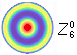Wavefront error in units of the peak aberration coefficient (S, C, A and P for lower-order spherical aberration, coma, astigmatism and defocus, respectively); ρ is the pupil radius normalized to 1 and θ is the pupil angle. Functions w/o asterisk give P-V wavefront error.  Functions with the asterisk give relative wavefront deviations form zero mean circle; they equal peak (i.e. one half P-V) wavefront error, except for balanced primary spherical aberration, where zero mean splits the P-V error in (2/3):(1/3) proportion, as shown below. For instance, for the peak aberration coefficient S=0.00055mm, the maximum negative deviation given by the peak wavefront aberration relation WP=(ρ42+1/6)S, is -1/12 (in units of 550nm wavelength, thus with S=1) for ρ=0.5, and the maximum positive deviation, for ρ=0 and ρ=1 is 1/6, for λ/4 P-V error (at paraxial focus, WP-V=Sρ4, or 1 wave).
 According to the convention, measuring radial angle θ from x+ toward y+ axis (same as on FIG. 30), with a positive superscript indicating cosine, and negative sine function.  This implies that wavefronts oriented with the meridional peak at 3PM clock position are in the cosine mode, and those with the meridional zero at the same point are in the sine angular frequency mode. As mentioned, angular differential in the angle of orientation between the two is given by 90/m, m being the superscript integer (for instance, going from cosine to sine function rotates wavefront by 90° for primary coma, and by 45° for primary and secondary astigmatism). Combining the variety of forms defined by polynomials makes possible modeling all types of asymmetric wavefront deviations.

Note that Zernike term Zi in columns 2 and 4 is often given in inappropriate small-letter notation (which is appropriate for denoting the coefficient), instead of the proper capital-letter notation; two-index notations at left (column 6, next to the wavefront map), relate Wyant's notation to the ANSI standard indexing scheme, with positive top index m indicating cosine, and negative indicating sine function.

Numerical value of the Zernike term Z is given by a product of its polynomial P for ρ=1 and θ=0 for polynomials containing pupil angle θ, normalization factor N and Zernike coefficient z, thus Z=PNz. And since P iz always 1 for ρ=1, Z=Nz. The Zernike coefficient z is nominally equal to the RMS wavefront error, except that it can be negative, as well as positive. Its sign is determined by the orientation of the wavefront point for ρ=1 (edge) relative to zero mean. For instance, primary spherical aberration shown on FIG. 32 could be either under- or overcorrection, depending on the direction of light (right-to-left and left-to-right, respectively). As shown, light travels right-to-left, making it undercorrection, and the coefficient is positive. For overcorrection it would have been negative. The sign is only relevant for orientation of the actual wavefront deviation (P-V), which can be obtained from the coefficient. The RMS wavefront error, equal to the coefficient in its absolute value (i.e. RMS=|z|), is always positive.

Following table shows the relation between the value of Zernike term (Z) and Zernike coefficient (z) to RMS and P-V wavefront error for primary and secondary aberrations, for the "diffraction limited" RMS wavefront error 0.0745 (hence for |z|=0.0745) in units of wave. Both Zernike term Z and coefficient z can be numerically positive or negative, so they are given as absolute values. Their sign is always the same. It does not affect the RMS error, which is always positive, but does determine the sign for the peak deviation from zero mean, i.e. indicates the direction of deviation with respect to zero mean.

 ABERRATION N |Z|=N|z| (|z|=0.0745) WFE Max. deviation from zero mean P-V Defocus √3 0.1291 0.1291 0.2582 Primary astigmatism √6 0.1826 0.1826 0.3652 Primary coma √8 0.2108 0.2108 0.4216 Balanced primary spherical √5 0.1666 0.1666 0.2500 Trefoil √8 0.2108 0.2108 0.4216 Secondary astigmatism √10 0.2357 0.2357 0.4714 Secondary coma √12 0.2582 0.2582 0.5164 Secondary spherical √7 0.1972 0.1972 0.3944

Value of the Zernike term reflects the maximum (along the axis of aberration) edge deviation from zero mean, also in units of wavelength. This maximum deviation is identical on both sides of zero mean (i.e. for the two opposite end points along the axis of aberration where the deviation, for ρ=1 and unit polynomial value is determined by cosθ or cos2θ - for the cosine term - thus equal in magnitude and opposite in sign for the extreme values of the trigonometric function) for all aberrations except primary spherical, where the maximum deviation on the opposite side of zero mean is half as large (as shown on FIG. 32; it can be found by determining the value of ρ for the maximum deviation on the opposite side of zero mean, for which the first derivative of 6ρ4-6ρ2+1 is zero). Thus the P-V error is 1.5N|z| for spherical aberration, and 2N|z| for all others.

These values also can be expressed in microns. For example, assuming 0.55μm wavelength, the corresponding values for defocus would have been |Z|=0.071μm for the term, and |z|=0.041μm for the coefficient. Obviously, Zernikes' value expressed in units of wavelength vary with the wavelength.

The combined P-V wavefront deviation for given set of Zernikes is a sum of the individual deviations for each term at every point of the wavefront. For instance, for the edge point above (ρ=1), it is given by the sum of all P-V values in the last column, with their sign being determined by that of the corresponding Zernike term. Combined RMS wavefront error for two or more Zernike terms is a square root of the sum of all the RMS wavefront errors individually squared.

3.5.2. Zernike aberrations    ▐    3.5.3. Zernike expansion schemes